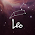## Wednesday, July 10, 2013

### Fast Fibonacci

I was looking for a faster Fibonacci algorithm and stumbled across this one. The doubling method assumes we know F(n) and F(n+1) to produce F(2n) and F(2n+1) out of the following formulas:

F(2n) = F(n) * (2*F(n+1) - F(n))
F(2n+1) = F(n+1)2 + F(n)2

The formulas can be extracted from the matrix exponentiation algorithm mentioned in the above article and reduced of redundant calculations.

Note:
Integer.numberOfLeadingZeros is a Java shortcut to get to the highest set bit. It may be implemented in some other way in different languages. It is needed here to avoid a few iterations; in our case considering we have 32 iterations and we can get an n up to 92, we can save up to 24 iterations.

1.2.